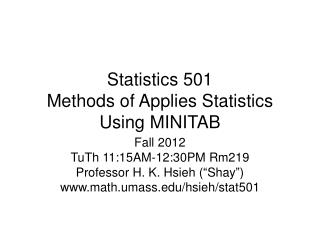Download PresentationStatistics 501 Methods of Applies Statistics Using MINITAB

# Statistics 501 Methods of Applies Statistics Using MINITAB - PowerPoint PPT PresentationDownload Presentation## Statistics 501 Methods of Applies Statistics Using MINITAB

- - - - - - - - - - - - - - - - - - - - - - - - - - - E N D - - - - - - - - - - - - - - - - - - - - - - - - - - -
##### Presentation Transcript

1. Statistics 501 Methods of Applies StatisticsUsing MINITAB Fall 2012 TuTh 11:15AM-12:30PM Rm219 Professor H. K. Hsieh (“Shay”) www.math.umass.edu/hsieh/stat501

2. Course Information • Instructor: Professor H. K. Hsieh (“Shay”) • Contact Information: LGRT 1444, (O) 545-1796; (Cell) 548-0798 • Email: hsieh@math.umass.edu • Texbook: Introduction to probability & Statistics, by Mendenhall, Beaver & Beaver, 13th edition, Brooks/Cole, 2009.

3. MINITAB Statistical Software • MINITAB software is available on most university public computers (e.g., in Tower Library basement). Minitab-16 is the latest version. • Minitab-16 is the latest version. • You can download to rent for 1 year (\$29.99) or purchase it perpetually (\$99.99) (if you want to use for your research work) • through www.onthehub.com/minitab/ • Free-trial is only for 30 days, and cannot be renewed on the same computer.

4. Requirements • Qualification: junior, senior or graduate students with logical thinking ability. Motivation to learn statistics is essential. • As the teaching is intensive, students are expected to attend all lectures if possible. • HW 30%, Test I 20%, Test II 20%, Final Exam 30%. Grade: A(95-100), A-(90-94), B+(86-89), B(81-85), B-(78-80), C+(75-77), C(71-74), C-(66-70), D+(61-65), D(56-60), F(0-55)

5. Syllabus • Statistical analysis using MINITAB with emphasises on identifying questions to be answered, • how to use MINITAB and interpret computer outputs: Exploratory data analysis - • population, sample, frequency distribution, empirical distribution, dot plots, stem and leaf • plots, histogram, quantiles, interquartile range, box plots, sample mean, sample variance; • Bivariate/multivariate Data - side-by-side box plots, scatter plots; matrix scatter plots;

6. Probability concepts -- sample space, events and their probabilities, random sampling • Random variables and their distributions, • Expected value and variance of a random variable • (discrete or continuous), binomial, hyper-geometric, and normal distributions • Sampling distribution -- random samples, statistic, sampling error • Sampling distribution of mean and proportion • Law of large numbers, Central limit theorem

7. Statistical Inference • Estimation of parameters – point estimation and interval estimation for means and proportions • Hypothesis testing for means and proportions -- one-sided, two-sided tests • one-sample t, two-sample t, paired t • Testing equality of variances;

8. Regression Analysis • Simple and multiple regression analysis • Correlation, least squares principles • Confidence interval for coefficients, mean response, single response • Logistic regression • Principal components analysis • Time series modeling

9. Analysis of variance • Testing equality of two or more population means, multiple comparisons • Two-factor ANOVA – testing for interactions, comparing means when interactions exist, and when interactions do not exist • Design by blocks

10. Analysis of categorical data • Contingency tables multinomial experiments, chi-square goodness-of-fit test • Tests based on ranks Wilcoxon rank-sum test, Wilcoxon signed rank test • Correlation based on ranks.

11. Home work sets • Write or type on 8x11 size paper • Write “Stat501” and your name on top of 1st page, and write and explain solutions according to problem number sequence • Referring your answers to computer outputs attached to the end of homework. • Computer output must be attached if computer is used for computation, otherwise no points will be creidted.

12. Staple up the papers. • Homework is expected to be turned in in time; • 20% off if turned in the 2nd day, 50% off if turned in 3rd day, and no point afterwards.